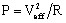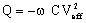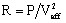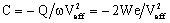# Example of a capacitor

## Capacitor with dielectric

Let us consider a Steady state AC Electric problem comprising a real capacitor and a voltage source applied at its terminals. The problem does not contain another voltage supply.

With:

• Veff, the rms value of the voltage applied to the capacitor, and ω the pulsation,
• the resistance R (in Ω) and the capacitance C (in F) of the equivalent RC parallel circuit of the capacitor. The dielectric material of the capacitor is supposed to be linear in order to enable the definition of the capacitance C.

## Capacitance and resistance of the capacitor

The complex apparent power S (in VA) of the capacitor has the expression: S = P + j Q

where:

• P is the active power (in W):• Q is the reactive power (in VAr):From the active power of the capacitor we can compute the equivalent resistance:From the active power of the capacitor we can compute the equivalent capacitance: